Create a new printableGeometry
Math Worksheets

Sample - Click above to make a new math worksheet (PDF).
 Name _____________________________Date ___________________
Volume and Surface Area
Fill in the missing values for a rectangular prism.
1.
 length 4 width 1 height ________ surface area ________ volume 28

 2 * This is a pre-made sheet.Use the link at the top of the page for a printable page.
3.
 length 13 width ________ height 9.6 surface area ________ volume 1048.32

Find the volume of each solid to the nearest tenth. (use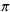= 3.14)
4.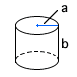a  = 9 in b  = 19 in

5.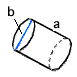a  = 19.7 m b  = 56.6 m

6.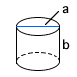a  = 2 km b  = 17 km

Find the volume of each solid to the nearest tenth. (use= 3.14)
7.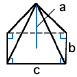a  = 9 ft b  = 8 ft c  = 7 ft

8.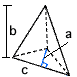a  = 1 km b  = 7 km c  = 6 km

9.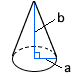a  = 3.3 cm b  = 8.5 cm

Find the volume of each solid to the nearest tenth. (use= 3.14)
 10 pyramid: B = 14 km2, h = 15 km
 11 cylinder: d = 8 m, h = 25 m

 Key #2
 aKey #2
Find the surface area of each solid to the nearest tenth. (use= 3.14)
12.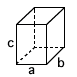a  = 8.6 mm b  = 8.2 mm c  = 9.6 mm

13.a  = 46 cm b  = 47 cm c  = 45 cm

14.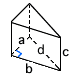a  = 33 in b  = 56 in c  = 8.5 in d  = 65 in

Find the surface area of each solid to the nearest tenth. (use= 3.14)
15.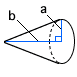a  = 4 in b  = 9.7 in

16.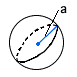a  = 12 mm

17.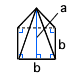a  = 4 cm b  = 19 cm c  = 19 cm

Complete.
 18 Captain Howard had his crew paint the smokestack on his ship the Sea Snail. The smokestack is shaped like a cylinder and is 35 feet 2 inches tall. The radius of the smokestack's base is seven feet. What is the volume of the smokestack?
 19 How much cement is needed to build a sidewalk that is six hundred fifty feet long, four feet wide and five inches thick? Round your answer to the nearest cubic foot.